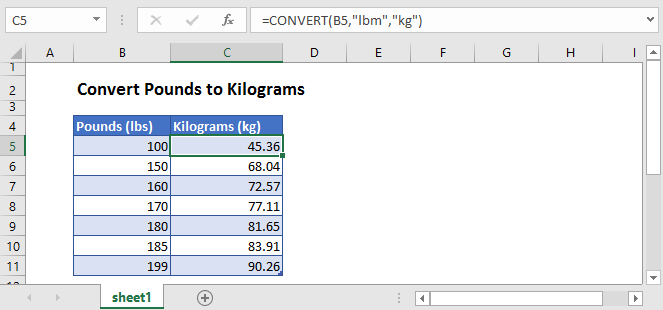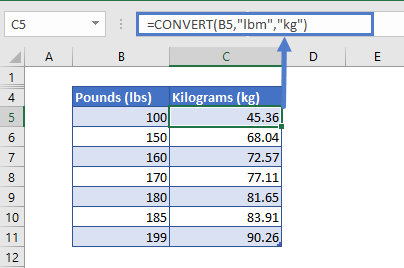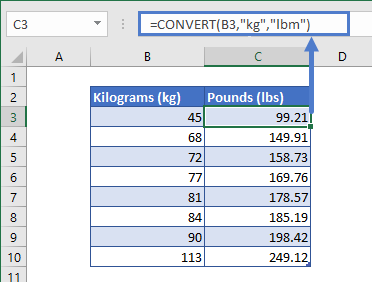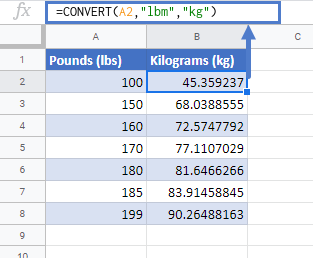# Convert Pounds to Kilograms in Excel & Google Sheets

This tutorial will demonstrate how to convert values given in pounds to kilograms (or kilograms to pounds) in Excel & Google Sheets.## Using the CONVERT Function

The CONVERT function is the best method to convert value given in pounds to kilograms.

Let’s test the above formula on the example for better understanding. Say in column B we have some weight measurements that need to be converted to kilograms. Type the following formula in cell C4 and drag it down:

``=CONVERT(B5,"lbm","kg")``## Convert Kilograms to Pounds

Just by switching the measurements arguments, we can convert the values given in kilograms to pounds:

``=CONVERT(B3,"kg","lbm")``## Convert Pounds to Kilograms in Google Sheets

The conversion of pounds to kilograms using the CONVERT Function works the same in Google Sheets as in Excel:### Excel Practice Worksheet

Practice Excel functions and formulas with our 100% free practice worksheets!